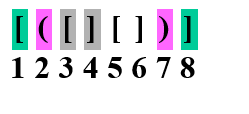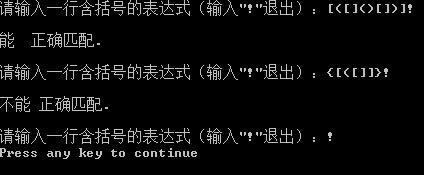• 请写一个程序，判断给定表达式中的括号是否匹配，既左右括号顺序和数量都匹配。 输入说明 输入为一个表达式字符串，长度不超过50。 输出说明 对输入的表达式，若其中的括号匹配的，则输出“yes”，否则输出“no...
问题描述
表达式中的合法括号为“(”、“)”、“[”、“]”、“{”、“}”，这三种括号可以按照任意的次序嵌套使用。
请写一个程序，判断给定表达式中的括号是否匹配，既左右括号顺序和数量都匹配。
输入说明
输入为一个表达式字符串，长度不超过50。
输出说明
对输入的表达式，若其中的括号是匹配的，则输出“yes”，否则输出“no”。
！！！注意，这个只有在英文输入法下有效，中文输入法下不当成括号（没有出现括号默认为yes）经过测试应该没啥问题
#include<stdio.h>//消消乐原理
#include<string.h>
int main()
{ char a;
int b=0,c=0,d=0,e=0,f=0,g=0;//定义几个数字
int i;
gets(a);
for(i=0;i<strlen(a);i++)//找括号
{ if(a[i]=='(')//如果是左括号，bcd+1，代表增加左括号一次
{b++;}
else if(a[i]=='[')
{c++;}
else if(a[i]=='{')
{d++;}
else if(a[i]==')')//如果是右括号，看看能不能与左括号消掉 ，
{if(b>0){b--;}//如果右括号前面有左括号，对应bcd-1；代表消掉一个左括号
else e++;//如果右括号前面一个左括号都没有，efg++，代表消不掉了
}
else if(a[i]==']')
{if(c>0){c--;}
else f++;
}
else if(a[i]=='}')
{if(d>0){d--;}
else g++;
}
}
if(b==0&&c==0&&d==0&&e==0&&f==0&&g==0)//如果都消掉了（包括左右括号匹配的情况）
{printf("yes");
}
else printf("no");

return 0;
}



展开全文• 数据结构习题与解析（B级第3版） 李春葆 喻丹丹 编著 3.2
• 假设表达式中允许括号嵌套，则检验括号是否匹配的方法可用“期待的急迫程度”这个概念来描述。 我们下面给和例子进行说明： 可能出现的不匹配的情况： ① 盼来的右括号不是所“期待”的； ② 到来的是“不速之客” ...
 原理说明算法思路代码演示
原理说明
假设表达式中允许括号嵌套，则检验括号是否匹配的方法可用“期待的急迫程度”这个概念来描述。
我们下面给和例子进行说明：可能出现的不匹配的情况：
① 盼来的右括号不是所“期待”的；
② 到来的是“不速之客”  （右括号多）；
③ 到结束也未盼来所“期待”的括号 （左括号多）。
算法思路
1）凡出现左括号，则进栈；
2）凡出现右括号，首先检查栈是否空。
若栈空，则表明该“右括号”多余；
否则和栈顶元素比较，
若相匹配，则“左括号出栈”，
否则表明不匹配。
3）表达式检验结束时，
若栈空，则表明表达式中匹配正确，
否则表明“左括号”有多余的。
代码演示
#include <stdio.h>
#include <string.h>
#include <malloc.h>

bool isValid(const char* s);

int main()
{
const char* tmp= "()[]{}";
if (isValid(tmp))
printf("ok\n");
else
printf("no\n");
return 0;
}
bool isValid(const char* s)
{
int len = strlen(s);
if (len % 2 != 0)
return false;

char tmp = 0;
char* stack = (char *)malloc(len * sizeof(char));
int pos = -1;
for (int i = 0; i < len; i++)
{
if (s[i] == '{' || s[i] == '[' || s[i] == '(')
{
pos++;
stack[pos] = s[i];
}
else
{

if (pos < 0)
return false;

tmp = stack[pos];
if (s[i] == '}')
{
if (tmp != '{')
return false;
else
pos--;
}

if (s[i] == ']')
{
if (tmp != '[')
return false;
else
pos--;
}

if (s[i] == ')')
{

if (tmp != '(')
return false;
else
pos--;
}
}
}
if (pos < 0)
return true;
return false;

}

运行结果展开全文• //顺序栈的使用举例：括号匹配 #include #include #define OK 1 #define ERROR 0 #define TRUE 1 #define FALSE 0 #define STACK_INIT_SIZE 100 #define STACKINCREMENT 10 #define BUFFERSIZE 256
//顺序栈的使用举例：括号的匹配
#include <stdio.h>
#include <stdlib.h>
#define OK      1
#define ERROR   0
#define TRUE    1
#define FALSE   0
#define STACK_INIT_SIZE 100
#define STACKINCREMENT 10
#define BUFFERSIZE 256
typedef int Status; //函数返回状态
typedef char SElemType;  //栈元素类型
typedef struct{//栈结构定义
SElemType *base;
SElemType *top;
int stacksize;
}SqStack;
Status InitStack(SqStack *S){
//构造一个空栈S
S->base=(SElemType *)malloc(STACK_INIT_SIZE*sizeof(SElemType));
if(!S->base)//分配失败
{
printf("分配内存失败.\n");
exit(0);
}
S->top=S->base;
S->stacksize=STACK_INIT_SIZE;
return OK;
}
Status DestroyStack(SqStack *S){
//销毁栈S，S不再存在
if(!S)//S为空
{
printf("指针为空，释放失败.\n");
exit(0);
}
free(S);
return OK;
}
Status ClearStack(SqStack *S){
//把栈S置为空栈
if(!S)//S不存在
return FALSE;
S->top=S->base;//直接将栈顶指针指向栈底
return OK;
}
Status StackEmpty(SqStack S){
//若栈S为空栈，则返回TRUE，否则返回FALSE
if(S.top==S.base)
return TRUE;
else
return FALSE;
}
int StackLength(SqStack S){
//返回S元素的个数，即栈的长度
return S.stacksize;
}
Status GetTop(SqStack S,SElemType *e){
//若栈不为空，则用e返回S的栈顶元素，并返回OK；否则返回FALSE
if(S.top==S.base){
//printf("栈为空.\n");
return FALSE;
}else{
*e=*(S.top-1);
//printf("%c",*e);
return OK;
}
}
Status Push(SqStack *S,SElemType e){
//插入元素e为新的栈顶元素
if(S->top-S->base>=S->stacksize){//栈已满，追加存储空间
S->base=(SElemType *)realloc(S->base,(S->stacksize+STACKINCREMENT)*sizeof(SElemType));
if(!S->base)
{
printf("重新申请空间失败.\n");
exit(0);
}
S->top=S->base+S->stacksize;//更改栈顶指针
S->stacksize+=STACKINCREMENT;
}
*S->top++=e;
return OK;
}
Status Pop(SqStack *S,SElemType *e){
//若栈S不为空，则删除S的栈顶元素，用e返回其值，并返回OK,否则返回ERROR
if(S->top==S->base){//栈为空
printf("栈为空.\n");
return ERROR;
}
*e=*(--S->top);
return OK;
}
Status StackTraverse(const SqStack *S){
//从栈底到栈顶依次对每个元素进行访问
SElemType *p=S->base;
if(S->base==S->top)
{
printf("栈为空.\n");
return FALSE;
}
//printf("栈中元素：");
while(p!=S->top)
{
printf("%c",*p++);
}
printf("\n");
return OK;
}
Status BracketMatch(SqStack *S,const char *string){
//输入字符串string中的括号匹配，返回TRUE；否则返回FALSE
const char *p=string;
SElemType e;
InitStack(S);
while(*p!='\0'){//遍历字符串
switch(*p){//判断p的值
case '('://左括号，入栈
case '[':
case '{':
Push(S,*p);
//printf("Push %c",*p);
break;
case ')':
if(FALSE==GetTop(*S,&e))
return FALSE;
if(e=='('){
if(ERROR==Pop(S,&e))
return FALSE;
//printf("Push %c",*p);
}else
return FALSE;
break;
case ']':
if(FALSE==GetTop(*S,&e))
return FALSE;
if(e=='['){
if(ERROR==Pop(S,&e))
return FALSE;
//printf("Push %c",*p);
}else
return FALSE;
break;
case '}':
if(FALSE==GetTop(*S,&e))
return FALSE;
if(e=='{'){
if(ERROR==Pop(S,&e))
return FALSE;
//printf("Push %c",*p);
}else
return FALSE;
break;
default:
;
}//switch
p++;
}//while
if(!StackEmpty(*S))//字符串遍历完，栈非空，不匹配
return FALSE;
return TRUE;
}
int main()
{
char *string;
SqStack stack;
string=(char*)malloc(sizeof(char)*BUFFERSIZE);
if(!string){
printf("分配内存失败.\n");
exit(0);
}
while(1){
printf("请输入一行含括号的表达式（输入\"!\"退出）：");
gets(string);
if(string=='!')//退出
break;
if(TRUE==BracketMatch(&stack,string)){
printf("\n能  正确匹配.\n\n");
}else{
printf("\n不能 正确匹配.\n\n");
}
}//while
return 0;}

运行结果：展开全文源代码
• //顺序栈的使用举例：括号匹配 //作者：nuaazdh //时间：2011年12月5日 #include #include #define OK 1 #define ERROR 0 #define TRUE 1 #define FALSE 0 #define STACK_INIT_SIZE 100 #define ...
//顺序栈的使用举例：括号的匹配
//作者：nuaazdh
//时间：2011年12月5日
#include <stdio.h>
#include <stdlib.h>

#define OK      1
#define ERROR   0
#define TRUE    1
#define FALSE   0
#define STACK_INIT_SIZE 100
#define STACKINCREMENT 10
#define BUFFERSIZE 256

typedef int Status; //函数返回状态
typedef char SElemType;  //栈元素类型
typedef struct{//栈结构定义
SElemType *base;
SElemType *top;
int stacksize;
}SqStack;

Status InitStack(SqStack *S);
//构造一个空栈S
Status DestroyStack(SqStack *S);
//销毁栈S，S不再存在
Status ClearStack(SqStack *S);
//把栈S置为空栈
Status StackEmpty(SqStack S);
//若栈S为空栈，则返回TRUE，否则返回FALSE
int StackLength(SqStack S);
//返回S元素的个数，即栈的长度
Status GetTop(SqStack S,SElemType *e);
//若栈不为空，则用e返回S的栈顶元素，并返回OK；否则返回FALSE
Status Push(SqStack *S,SElemType e);
//插入元素e为新的栈顶元素
Status Pop(SqStack *S,SElemType *e);
//若栈S不为空，则删除S的栈顶元素，用e返回其值，并返回OK,否则返回ERROR
Status StackTraverse(const SqStack *S);
//从栈底到栈顶依次对每个元素进行访问
Status BracketMatch(SqStack *S,const char *string);
//输入字符串string中的括号匹配，返回TRUE；否则返回FALSE

int main()
{
char *string;
SqStack stack;
string=(char*)malloc(sizeof(char)*BUFFERSIZE);
if(!string){
printf("分配内存失败.\n");
exit(0);
}
while(1){
printf("请输入一行含括号的表达式（输入\"!\"退出）：");
gets(string);
if(string=='!')//退出
break;
if(TRUE==BracketMatch(&stack,string)){
printf("\n能  正确匹配.\n\n");
}else{
printf("\n不能 正确匹配.\n\n");
}
}//while
return 0;
}

Status InitStack(SqStack *S){
//构造一个空栈S
S->base=(SElemType *)malloc(STACK_INIT_SIZE*sizeof(SElemType));
if(!S->base)//分配失败
{
printf("分配内存失败.\n");
exit(0);
}
S->top=S->base;
S->stacksize=STACK_INIT_SIZE;
return OK;
}

Status DestroyStack(SqStack *S){
//销毁栈S，S不再存在
if(!S)//S为空
{
printf("指针为空，释放失败.\n");
exit(0);
}
free(S);
return OK;
}

Status ClearStack(SqStack *S){
//把栈S置为空栈
if(!S)//S不存在
return FALSE;
S->top=S->base;//直接将栈顶指针指向栈底
return OK;
}

Status StackEmpty(SqStack S){
//若栈S为空栈，则返回TRUE，否则返回FALSE
if(S.top==S.base)
return TRUE;
else
return FALSE;
}

int StackLength(SqStack S){
//返回S元素的个数，即栈的长度
return S.stacksize;
}

Status GetTop(SqStack S,SElemType *e){
//若栈不为空，则用e返回S的栈顶元素，并返回OK；否则返回FALSE
if(S.top==S.base){
//printf("栈为空.\n");
return FALSE;
}else{
*e=*(S.top-1);
//printf("%c",*e);
return OK;
}
}

Status Push(SqStack *S,SElemType e){
//插入元素e为新的栈顶元素
if(S->top-S->base>=S->stacksize){//栈已满，追加存储空间
S->base=(SElemType *)realloc(S->base,(S->stacksize+STACKINCREMENT)*sizeof(SElemType));
if(!S->base)
{
printf("重新申请空间失败.\n");
exit(0);
}
S->top=S->base+S->stacksize;//更改栈顶指针
S->stacksize+=STACKINCREMENT;
}
*S->top++=e;
return OK;
}

Status Pop(SqStack *S,SElemType *e){
//若栈S不为空，则删除S的栈顶元素，用e返回其值，并返回OK,否则返回ERROR
if(S->top==S->base){//栈为空
printf("栈为空.\n");
return ERROR;
}
*e=*(--S->top);
return OK;
}

Status StackTraverse(const SqStack *S){
//从栈底到栈顶依次对每个元素进行访问
SElemType *p=S->base;
if(S->base==S->top)
{
printf("栈为空.\n");
return FALSE;
}
//printf("栈中元素：");
while(p!=S->top)
{
printf("%c",*p++);
}
printf("\n");
return OK;
}

Status BracketMatch(SqStack *S,const char *string){
//输入字符串string中的括号匹配，返回TRUE；否则返回FALSE
const char *p=string;
SElemType e;
InitStack(S);
while(*p!='\0'){//遍历字符串
switch(*p){//判断p的值
case '('://左括号，入栈
case '[':
case '{':
Push(S,*p);
//printf("Push %c",*p);
break;
case ')':
if(FALSE==GetTop(*S,&e))
return FALSE;
if(e=='('){
if(ERROR==Pop(S,&e))
return FALSE;
//printf("Push %c",*p);
}else
return FALSE;
break;
case ']':
if(FALSE==GetTop(*S,&e))
return FALSE;
if(e=='['){
if(ERROR==Pop(S,&e))
return FALSE;
//printf("Push %c",*p);
}else
return FALSE;
break;
case '}':
if(FALSE==GetTop(*S,&e))
return FALSE;
if(e=='{'){
if(ERROR==Pop(S,&e))
return FALSE;
//printf("Push %c",*p);
}else
return FALSE;
break;
default:
;
}//switch
p++;
}//while
if(!StackEmpty(*S))//字符串遍历完，栈非空，不匹配
return FALSE;
return TRUE;
}
展开全文c 语言 string struct 存储
• 顺序栈的基本操作 个人理解：顺序栈其实就是一个数组，只不过人为地给其加上限制，使得入栈元素满足后进先出的规则。在实际运用中，栈大都作为辅助结构，是帮助解决问题的一种手段。在考研中，单独对栈的编程题也很...数据结构
• 文章目录一、题目二、算法思想三、代码四、测试结果总结 一、题目 C语言里算术表达式中的括号只有小括号。 编写算法，判断 一个表达式中的括号是否正确配对，...若top==-1,则括号匹配； 若top！=-1,则括号不匹.数据结构 正则表达式
• （基于C语言的数据结构）顺序栈的基本操作和简单习题（数值转换、括号匹配）持续更新中 stack.h #include <stdio.h> #include <stdlib.h> #define STACK_INIT_SIZE 100 #define STACKINCREMENT 10 #...数据结构 stack
• 二、方便起见，这里我们使用顺序存储结构来存储待匹配的元素： 因为存储的是括号，所以数据部分是char类型。 我们开辟了10个连续的char型空间存储元素，1个Int型空间存储指针，指针的值为数组下标。 #include<...
• ① 相等 = 左括号遇到右括号相等说明左右括号匹配。 ① # 和 # 遇到的时候也是 = ，我们在表达式的左边人为的加上一个 # ,在表达式扫描完成之后再加上一个 # 表示表达式扫描完了。 ③ 上面表格中出现的空白部分表示...
• //用顺序栈实现括号匹配 #include<stdio.h> #define MAXSIZE 10 typedef int bool; #define true 1 #define false 0 typedef struct{ char data[MAXSIZE]; //静态数组存放栈中元素 int top数据结构
• 3利用的操作实现括号匹配的检验。 ．括号匹配算法思想 （1）出现的凡是“左括号”，则进栈； （2）出现的是“右括号”， 首先检查是否空？ 若空，则表明该“右括号”多余 否则和栈顶元素比较？ 若相...
• //括号匹配问题中的应用，假设表达式中允许存在三种括号：方括号和圆括号和花括号，其嵌套顺序任意，即([](){})或[{([][])}]等均为正确格式，[(])或([))或(()]均为不正确格式 #include&lt;stdio.h&gt; #...
• 利用顺序栈的操作函数 typedef char SElemType; #include"Stack.h" int kuohaopipei() { char p; SqStack s; char temp; InitStack(s); p=getchar(); while(p!='\n') { if(p=='('||p=='['||p=='{') Push(s,...数据结构
• typedef struct//顺序栈 { char data[MaxSize]; int top; }SqStack; bool StackEmpty(SqStack &S)//判断栈空 { if(S.top==-1) return true; else return false; } bool Push(SqStack &S,char x)...
• 计算机是如何检查括号匹配的： 下面这几种情况会被判定为是非法的： （1）括号形状不匹配，则后面的就都不用看了： （2）出现了一个单身的右括号，也就是空了，没有左括号出来和它配对了： （3）出现了左括号...
• C语言数组实现括号匹配 废话不多说，直接上代码 #include<iostream> using namespace std; int Match(char *str) { char s; //定义顺序栈，假定最长有效字符为100 int top = -1; //初值指针-1 for(int...数据结构
• 已知以字符型顺序表表示的表达式含有三种扩号“（”、“）”、“ “[” 、“]” 、“{” 和“}” ，它们可嵌套使用，试写出算法判断给定表达式中所含扩号是否正确配对出现。 代码实现 #include<stdio.h>...数据结构
• 文 | 猿天罡本题源自LeetCode，题号20。...左括号必须以正确的顺序闭合。注意空字符串可被认为是有效字符串。示例 1:输入: "()"输出: true示例 2:输入: "()[]{}"输出: true示例 3:输入: "(]"输出...
• 问题：假设表达式中允许包含三种括号，圆括号括号括号，嵌套顺序随意。 前提准备 #include <stdio.h> #include <stdlib.h> #define MAXSIZE 100 #include <string.h> #define NOMATCH -1//...算法 数据结构
• 括号必须以正确的顺序闭合。 代码 void bracketmatch(){ char *str = (char*)malloc(sizeof(char)*10); printf("请输入要匹配括号组："); fflush(stdout);//清空输出缓冲区（否则我上面打印不出来） scanf...数据结构
• 数制转换：将十进制数转换为任意进制数。由数制转换的计算公式 N = (N / d) × d + N % d (其中N为想转换...括号匹配：即利用检验输入的括号是否正确配对，包括了三种括号的匹配，即( )、[ ]、{ }。其嵌套的顺序随...
• 顺序栈的应用（一） ： 括号匹配问题 问题：C语言的算术表达式中的括号只有小括号，编写一个算法判断一个表达式中的括号是否配对？ 算术表达式已存入数组exp[]中，表达式中字符的个数为 n */ //判断数组中...数据结构# c语言括号匹配顺序栈c语言 订阅